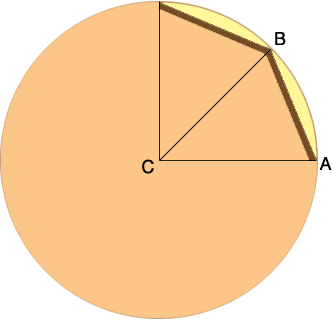SEARCH HOMEMath Central Quandaries & QueriesQuestion from Cassie: I'm trying to construct a Wishing Well made of treated 2x4 wood, so the actual measurement is 3 1/2 by 1 1/2. The well is going to be three feet in diameter. I'm trying to construct this as circular as possible, what angle should the wood panels be cut at?Suppose the wishing well has n sides. I drew a picture assuming n = 8 and I'll use it to develop a formula for the angle that will work for any n.Since there are n sides the measure of the angle BCA is 360/n degrees. The measures of the angles CAB and ABC are equal and the sum of the angles of a triangle is always 180 degrees and hence the measure of the angle ABC is

1/2 (180 - 360/n).

This simplifies to

angle ABC = 90 - 180/n degrees.

Thus for example if n = 8 then the measure of the angle ABC is 90 - 180/8 = 90 - 22.5 = 67.5 degrees.

I hope this helps,
PennyMath Central is supported by the University of Regina and The Pacific Institute for the Mathematical Sciences.